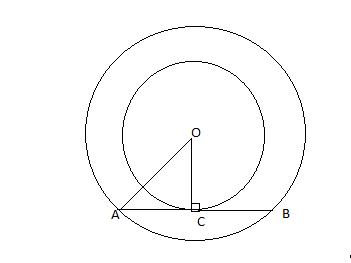QUESTION

# In the given figure below, two concentric circles of radii a and b (a>b) are given. The chord AB of larger circle touches the smaller circle at C. The length of AB is A. $\sqrt {{a^2} - {b^2}}$ B. $\sqrt {{a^2} + {b^2}}$ C. $2\sqrt {{a^2} - {b^2}}$D. $2\sqrt {{a^2} + {b^2}}$In $\Delta$AOC,
$O{A^2} = O{C^2} + A{C^2}$
$A{C^2} = O{A^2} - O{C^2} \\ A{C^2} = {a^2} - {b^2} \\ AC = \sqrt {{a^2} - {b^2}} \\$
AB=2$AC$=2$\sqrt {{a^2} - {b^2}}$
Here the answer is $2\sqrt {{a^2} - {b^2}}$